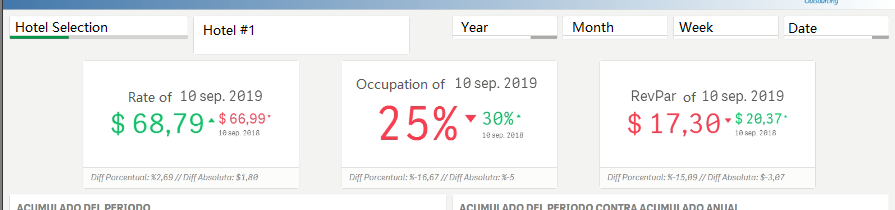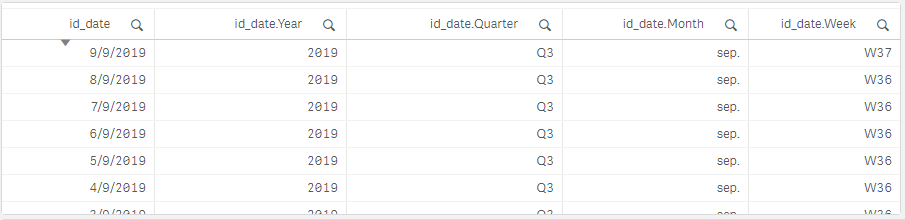# New to Qlik Sense

Discussion board where members can get started with Qlik Sense.

New Contributor

## How to create a fixed KPI with set analysis

Hello everyone!

I'm new with Qlik Sense and I'm doing my first Dashboard where I need to create a fixed KPI,  where the last Rate of the date has to be displayed. If you select one of the 3 hotels (each having their own Rate)  it should show the latest Rate from this year (11/09/2019) and previous year to compare it ( 11/09/2018).The Rate number has two types of data, History and OTB and I need to show only the first one, the History rate. The id_date have all te dates of the tables, this is the one i'm using to filter through time.For the previous KPI's I'm using this expression:

Sum ({<id_date={"\$(=Date(max(id_date)))"}> * \$<hist_y_otb={"History"}>} Rate)

I don't know what I'm missing, whenever I make a selection it changes the values of the KPI´s Rate.  Im using the Date(max())  but in other posts from this forum, they recommend to use Date(Now()) or Today(date()).

I also need to show the last year's Rate fixed value to compare it, but I'm having trouble with my expression:

Sum({<id_date={'\$(=Date(max(id_date)-365))'}> * \$<hist_y_otb={'History'}>} Rate)

Please! Can someone help me with this confusion of mine? thanks a lot!

Labels (5)

• ### Set Analisys

1 Solution

Accepted SolutionsEmployee

## Re: How to create a fixed KPI with set analysis

Hi,

For your first expression, you could simplify a little:
Sum ({\$<id_date={"\$(=Date(max(id_date)))"},hist_y_otb={History}>} Rate)

To stop it from changing based on selections, just add those fields you want to ignore selection in as well:
Sum ({\$<id_date={"\$(=Date(max(id_date)))"},hist_y_otb={History}, id_date.Year=, id_date.Quarter=,id_date.Month=,id_date.Week=>} Rate)

or if you want to ignore ALL selections, then use 1 instead of \$:
Sum ({1<id_date={"\$(=Date(max(id_date)))"},hist_y_otb={History}>} Rate)

For the same date previous year you could try:
Sum ({1<id_date={"\$(=max(id_date)-365"},hist_y_otb={History}>} Rate)Employee

## Re: How to create a fixed KPI with set analysis

Hi,

For your first expression, you could simplify a little:
Sum ({\$<id_date={"\$(=Date(max(id_date)))"},hist_y_otb={History}>} Rate)

To stop it from changing based on selections, just add those fields you want to ignore selection in as well:
Sum ({\$<id_date={"\$(=Date(max(id_date)))"},hist_y_otb={History}, id_date.Year=, id_date.Quarter=,id_date.Month=,id_date.Week=>} Rate)

or if you want to ignore ALL selections, then use 1 instead of \$:
Sum ({1<id_date={"\$(=Date(max(id_date)))"},hist_y_otb={History}>} Rate)

For the same date previous year you could try:
Sum ({1<id_date={"\$(=max(id_date)-365"},hist_y_otb={History}>} Rate)